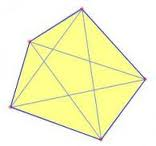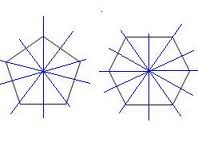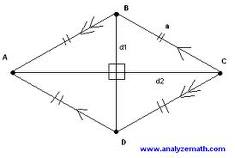## Monday, October 25

### Form 1 Mathematics Chapter 10

Form 1
Mathematics
Chapter 10
Polygons

1. Polygons 的种类：
a. Triangle            3边
b. Quadrilateral    4边
c. Pentagon          5边
d. Hexagon           6边
e. Heptagon          7边
f. Octagon             8边
g. Nonagon           9边
h. Decagon           10边

2. diagonals，对角线，就是把里面角的数量以直线划去别的角3. Symetry lines 就是对称轴，就像镜子找出来的一样4. 三角形的种类：
a. Acute-angled —— 三个角度少过90°，锐角三角形
b. Obtused-angled —— 一个角度超过90°，钝角三角形
c. Right angled —— 一个90°，两个45°，直角三角形
d. Isoceles —— 两个角度和长度一样，等边三角形
e. Equilateral —— 三个角度和长度一样，90°，等边三角形
f. Scalene —— 三个角度都不一样，不等边三角形

5. 四角形的种类：
a. Parallelogram —— ∠A=∠C, ∠D=∠Bb. Rectangle —— ∠A=∠B=∠C=∠D=90°，AB=CD，AC=BDc. Square —— AB=BC=CD=DA，∠A=∠B=∠C=∠D=90°d. Rhombus —— AB=BC=CD=DA，∠A∠B∠C∠D=90°e. Trapezium —— DC is parallel to AC6. 三角形和四角形的特征：

a. ∠A+∠B+∠C=180°b. ∠1+∠2=∠3c. ∠A+∠B+∠C+∠D=180°